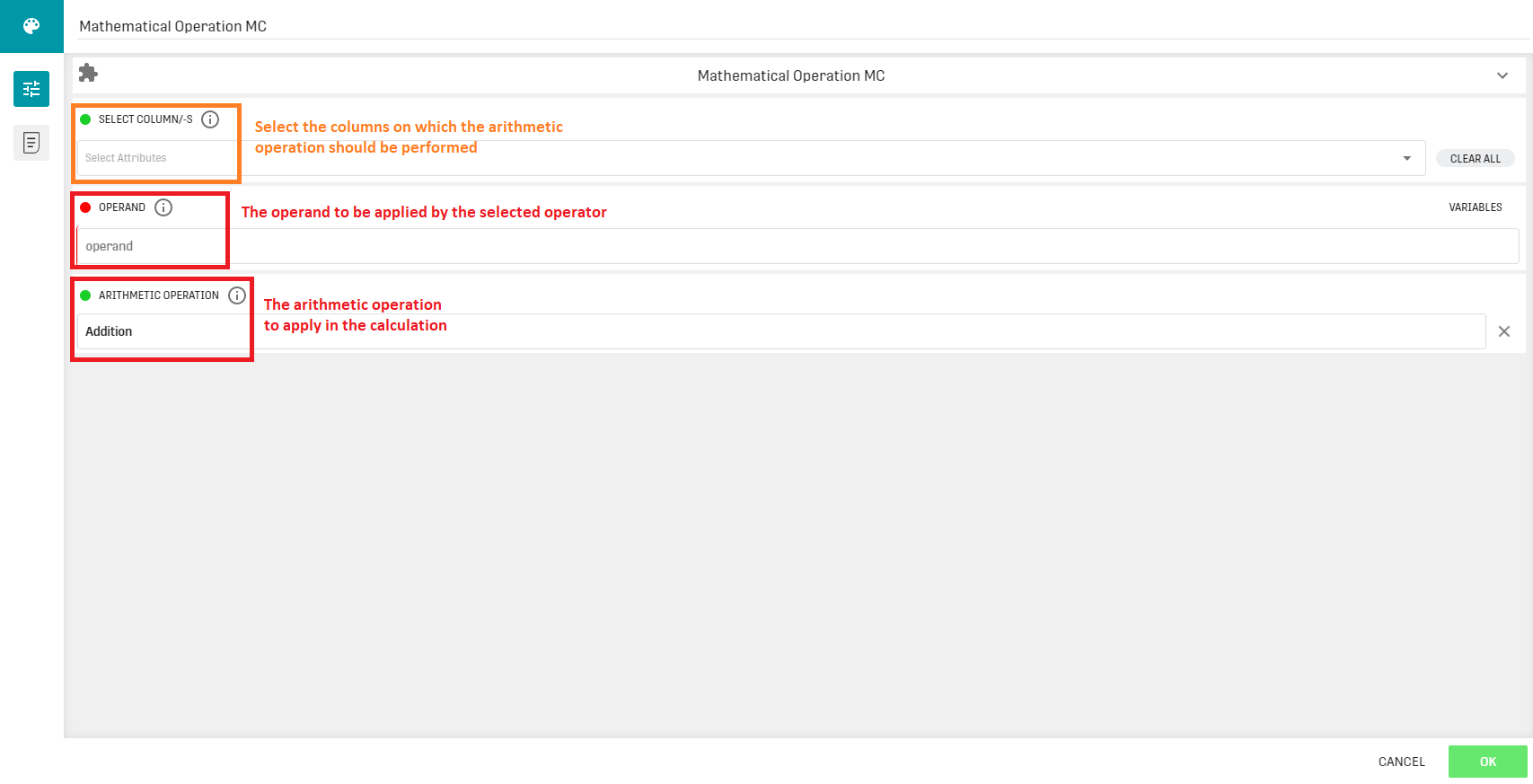# Mathematical Operation MC Processor

Modified on Tue, 30 Nov 2021 at 02:13 PM

# Overview

The Mathematical Operation MC (Multiple Column) Processor executes an arithmetic operation on the input columns and overwrites them with the result.

# Input

The processor requires at least one column of a numerical datatype as input.

# ConfigurationNote that the columns that haven't been selected in the first configuration field are also forwarded in the output without being altered. If no column is selected, then the operation is applied to all input columns.

# Output

The input table is forwarded as output, with the selected columns overwritten by the result of the mathematical operation.

# Related Articles

Mathematical Operation SC Processor

Extended Mathematical Operation Processor

Mathematical Timestamp Operation Processor

Mathematical Column Operation Processor